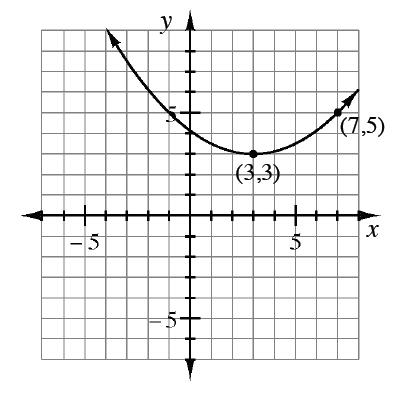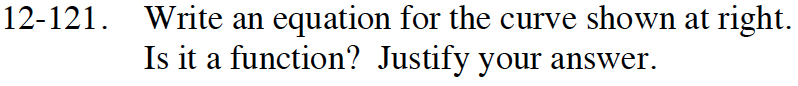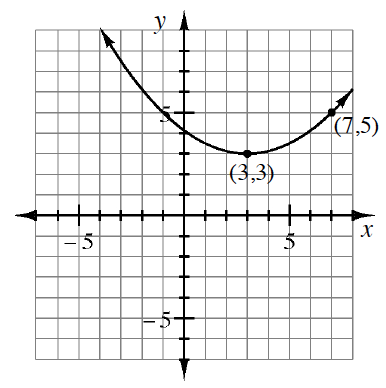Home > CCA2 > Chapter 12 > Lesson 12.2.2 > Problem12-121

12-121.

Write an equation for the curve shown below. Is it a function? Justify your answer. Homework Help ✎What parent graph does this look similar to?
What would the equation be?
And how do you determine if something is a function?

One possible answer isIs this a function?